# Star of a function element

(diff) ← Older revision | Latest revision (diff) | Newer revision → (diff)

Mittag-Leffler star

A star-like domain in which the given element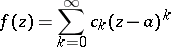of an analytic function (cf. Analytic function, element of an) can be continued analytically along rays issuing from the centre. The star consists of those points of the complex-plane which can be reached by analytic continuation ofas a power series along all possible rays from the centreof the series. If,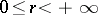, is a ray on which there are points that cannot be reached this way, then there is a point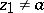on the ray such that the element can be continued to any point of the interval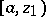but not beyond. If continuation is possible to any point of the ray, one puts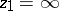. The set of points belonging to all intervalsis a (simply-connected) star-like domain about, called the star of the function element and denoted by. Analytic continuation in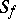results in a regular analytic function, which is the univalent branch inof the complete analytic function generated by the given element.

All points of the boundary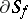are accessible (cf. Attainable boundary point). In questions of analytic continuation (see also Hadamard theorem) one also defines angular, attainable and well-attainable points of. A pointis called an angular boundary point of the star of a function element if its modulus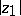is minimal among all points ofwith the same argument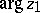. A point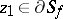is called an attainable boundary point of the star if there is a half-disc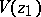such thatis regular everywhere insideand at the points of its diameter other than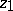. The point is said to be well-attainable if there is a sectorwith apexand angle greater than, such thatis regular in the domain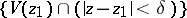for sufficiently small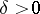.

G. Mittag-Leffler

showed that a regular functioncan be expressed in its star as a series of polynomials convergent inside: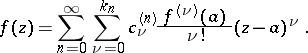(*)

Formula (*) is known as the Mittag-Leffler expansion in a star. The degrees of the polynomials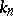and their coefficients,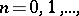are independent of the form ofand can be evaluated once and for all. This was done by P. Painlevé (see , ).

How to Cite This Entry:
Star of a function element. Encyclopedia of Mathematics. URL: http://encyclopediaofmath.org/index.php?title=Star_of_a_function_element&oldid=12951
This article was adapted from an original article by E.D. Solomentsev (originator), which appeared in Encyclopedia of Mathematics - ISBN 1402006098. See original article